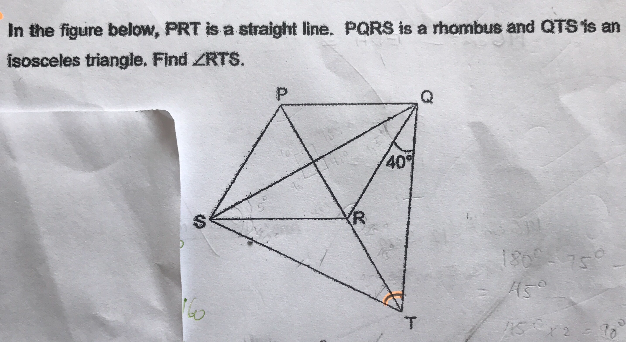# QuestionSource: Raffles Girls’ Primary

In the figure below, PRT is a straight line. PQRS is a rhombus and QTS is an isoceles triangle. Given that angle SQR = 30 degrees, (I believe this piece of information is missing from the diagram) find angle RTS.30 x 2 = 60 (angles RQS + RSQ)
180 – 60 = 120 (angle QRS)
360 – 120 = 240 (reflex angle QRS)
240/2 = 120 (angle SRT)
120 + 40 = 160
180 – 160 = 20

Ans : 20 degrees.

Hi can explain? Don’t understand still.